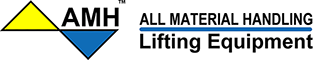Plate Lifting Clamps | 48WS

# Ecommerce Websites, Catalogs, and Marketing Services

## We Do Not Sell Products

BROWSE CATEGORIES

Search:

# Plate Lifting Clamps

## All Materials Handling, Inc.SKUInfo/Size
PCUA005
WLL Metic Tonne = 0.5, Weight (lbs) = 4.4, Plate Size A (in) = 0-5/8, B (in) = 1.7, C (in) = 4.2, D (in) = 8.5, E (in) = 1.2, F (in) = 0.4, G (in) = 2
PCUA010
WLL Metic Tonne = 1, Weight (lbs) = 9.9, Plate Size A (in) = 0-3/4, B (in) = 2.5, C (in) = 5.7, D (in) = 11.5, E (in) = 1.9, F (in) = 0.5, G (in) = 2.6
PCUA020
WLL Metic Tonne = 2, Weight (lbs) = 16.1, Plate Size A (in) = 0-1, B (in) = 3.1, C (in) = 6.9, D (in) = 14.5, E (in) = 2.7, F (in) = 0.6, G (in) = 3
PCUA030
WLL Metic Tonne = 3, Weight (lbs) = 33, Plate Size A (in) = 0-1 1/8, B (in) = 3.3, C (in) = 8.1, D (in) = 16.9, E (in) = 2.9, F (in) = 0.8, G (in) = 4
PCUA050
WLL Metic Tonne = 5, Weight (lbs) = 47.5, Plate Size A (in) = 0-2, B (in) = 3.9, C (in) = 9.4, D (in) = 18.9, E (in) = 3.1, F (in) = 0.9, G (in) = 4.3
Quick Overview
Call Mon. through Fri. 8:00 AM - 4:00 PM PST
Phone: 714-573-4083
Email: sales@48ws.com

Can't find what you want? Give us a call and we can help you find it.
Details
All steel body for durable strength in a smaller size.. Individually serialized and tested to 2 x WLL. Factory test certification of proof load is included.. For use with materials having surface hardness < HRC 37. 180° turning range for easy positioning or transfer of plates.. Lever insures pre-tensioning and release of grip jaws.. Replacement parts kits are available.. Meets: EU Directives: 2006/42/EC and ASME B30.20.